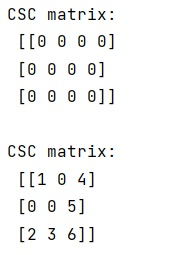# Scipy Sparse Arrays

Learn about the scipy sparse arrays or csc matrix in Python.
Submitted by Pranit Sharma, on February 20, 2023

NumPy is an abbreviated form of Numerical Python. It is used for different types of scientific operations in python. Numpy is a vast library in python which is used for almost every kind of scientific or mathematical operation. It is itself an array which is a collection of various methods and functions for processing the arrays.

Scipy sparse csc matrix is a Compressed Sparse Column matrix. This can be initiated in several ways:

• csc_matrix(D)- uses a dense matrix
• csc_matrix(S)- uses another sparse matrix
• csc_matrix((M, N), [dtype])- to construct an empty matrix with shape (M, N) dtype is optional, defaulting to dtype='d'.
• csc_matrix((data, (row_ind, col_ind)), [shape=(M, N)])-where data, row_ind and col_ind satisfy the relationship a[row_ind[k], col_ind[k]] = data[k].

Let's understand with the help of an example,

## Python code to demonstrate the example of scipy sparse arrays

```# Import numpy
import numpy as np

# Importing scipy sparse car matrix
from scipy.sparse import csc_matrix

# Creating a csc matrix
res = csc_matrix((3, 4), dtype=np.int8).toarray()

# Display result
print("CSC matrix:\n",res,"\n")

# Assigning elements
row = np.array([0, 2, 2, 0, 1, 2])
col = np.array([0, 0, 1, 2, 2, 2])
data = np.array([1, 2, 3, 4, 5, 6])
res = csc_matrix((data, (row, col)), shape=(3, 3)).toarray()

# Display matrix
print("CSC matrix:\n",res)
```

Output: